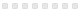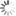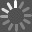MATLAB STATISTICS

Book - 2014
Rate this:MATLAB is a high-level language and environment for numerical computation, visualization, and programming. Using MATLAB, you can analyze data, develop algorithms, and create models and applications. The language, tools, and built-in math functions enable you to explore multiple approaches and reach a solution faster than with spreadsheets or traditional programming languages, such as C/C++ or Java.

MATLAB Statistics introduces you to MATLAB and begins by showing you how to use the Basic Module of MATLAB statistical functions, from the elementary mathematical functions, to special mathematical functions and the functions you can use in MATLAB to perform data analysis and basic statistical analysis.

You're then introduced to the MATLAB Statistics Toolbox, which extends the basic statistical functions of MATLAB, and you'll find practical hands-on examples throughout the book on how to use these extended MATLAB functions for descriptive statistics, probabilistic models, discrete and continuous random variables, confidence intervals and hypothesis contrasts, models of linear and non-linear regression, multivariate data analysis, quality control, design of experimentation and other content of industrial statistics.

What you'll learn How to use the MATLAB environment - with hands-on examples throughout the book How to work with the MATLAB Basic Module of statistical functions Using elementary mathematical functions with MATLAB How to perform data analysis and basic statistical methods with MATLAB How to work with the MATLAB Statistics Toolbox for extended statistic functions Descriptive statistics with MATLAB Probabilistic models in MATLAB How to work with discrete and continuous random variables in MATLAB Confidence intervals and hypothesis contrasts MATLAB approaches to models of linear and non-linear regression Multivariate data analysis with MATLAB Industrial statistics - how to approach quality control, and design of experimentation Who this book is for

This handy desktop reference is for people in a wide range of jobs that utilize statistics, or for academic pursuits, including researchers and students. It teaches how to use the most widely used statistical techniques in MATLAB to solve problems without being burdened with theory.

Publisher: Springer-Verlag New York Inc 2014
ISBN: 9781484202999
1484202996Opinion

From the criticsCommunity Activity

Comment

There are no comments for this title yet.

Age

There are no ages for this title yet.

Summary

There are no summaries for this title yet.

Notices

There are no notices for this title yet.

Quotes

There are no quotes for this title yet.Explore FurtherRecommendationsLoading...
No similar edition of this title was found at SLPL.

Try searching for MATLAB STATISTICS to see if SLPL owns related versions of the work.Loading...
[]
[]